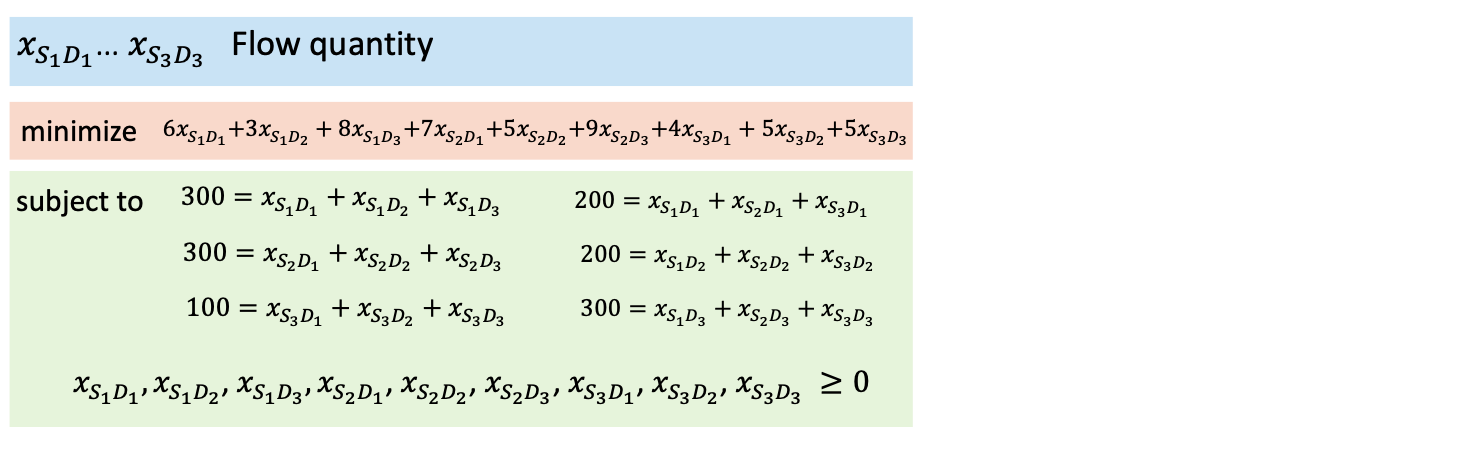# Notebook 3.2 - Transportation Problem (Explicit) using PuLP¶

We are now going to demonstrate the use of PuLP with a slightly larger problem. For this notebook, we will be considering the explicit transportation model formulation that we discussed in Part 2 of our session.

Since we pip-installed pulp while executing our previous notebook, there is no reason to repeat the process again.Our first step will be to import PuLP and initialise a model instance. As this is a minimisation, we are again using the LpProblem() function to initialise our model.

In :
from pulp import *

prob = LpProblem('problem',LpMinimize)


On this particular formulation, we are being given a lower-bound value for each decision variable, given the presence of the non-negativity constraints. We will therefore use the lowBound parameter when initialising each variable.

In :
x_s1d1 = LpVariable("x_s1d1", lowBound = 0)
x_s1d2 = LpVariable("x_s1d2", lowBound = 0)
x_s1d3 = LpVariable("x_s1d3", lowBound = 0)
x_s2d1 = LpVariable("x_s2d1", lowBound = 0)
x_s2d2 = LpVariable("x_s2d2", lowBound = 0)
x_s2d3 = LpVariable("x_s2d3", lowBound = 0)
x_s3d1 = LpVariable("x_s3d1", lowBound = 0)
x_s3d2 = LpVariable("x_s3d2", lowBound = 0)
x_s3d3 = LpVariable("x_s3d3", lowBound = 0)


We now begin populating our optimization problem.

Our objective function is:

$6x_{S1D1}+3x_{S1D2}+8x_{S1D3}+7x_{S2D1}+5x_{S2D2}+9x_{S2D3}+4x_{S3D1}+5x_{S3D2}+5x_{S3D3}$

In :
prob += 6 * x_s1d1 + 3 * x_s1d2 + 8 * x_s1d3 + 7 * x_s2d1 + 5 * x_s2d2 + 9 * x_s2d3 + 4 * x_s3d1 + 5 * x_s3d2 + 3 * x_s3d3


We can now add the constraints.

In :
prob += x_s1d1 + x_s1d2 + x_s1d3 == 300
prob += x_s2d1 + x_s2d2 + x_s2d3 == 300
prob += x_s3d1 + x_s3d2 + x_s3d3 == 100
prob += x_s1d1 + x_s2d1 + x_s3d1 == 200
prob += x_s1d2 + x_s2d2 + x_s3d2 == 200
prob += x_s1d3 + x_s2d3 + x_s3d3 == 300


You might not be able to tell by simply reading this notebook, but typing all these equations is incredibly tedious and repetitive.

It is also a very error-prone process - it took me 3 attempts to type the model correctly, as I had made several mistakes in quite a few variable names.

We can now print the model formulation to check that it corresponds to the one that we were trying to implement.

In :
print(prob)

problem:
MINIMIZE
6*x_s1d1 + 3*x_s1d2 + 8*x_s1d3 + 7*x_s2d1 + 5*x_s2d2 + 9*x_s2d3 + 4*x_s3d1 + 5*x_s3d2 + 3*x_s3d3 + 0
SUBJECT TO
_C1: x_s1d1 + x_s1d2 + x_s1d3 = 300

_C2: x_s2d1 + x_s2d2 + x_s2d3 = 300

_C3: x_s3d1 + x_s3d2 + x_s3d3 = 100

_C4: x_s1d1 + x_s2d1 + x_s3d1 = 200

_C5: x_s1d2 + x_s2d2 + x_s3d2 = 200

_C6: x_s1d3 + x_s2d3 + x_s3d3 = 300

VARIABLES
x_s1d1 Continuous
x_s1d2 Continuous
x_s1d3 Continuous
x_s2d1 Continuous
x_s2d2 Continuous
x_s2d3 Continuous
x_s3d1 Continuous
x_s3d2 Continuous
x_s3d3 Continuous



It all appears to be correct, and we can now solve oue problem instace using the solve() command.

In :
status = prob.solve()

print(f'STATUS\n{LpStatus[status]}\n')

STATUS
Optimal



As before, we are using the following block of code to print the result of our model.

In :
print('SOLUTION:')
for v in prob.variables():
print(f'\t\t{v.name} = {v.varValue}')

print('\n') # Prints a blank line
print(f'OBJECTIVE VALUE: {prob.objective.value()}')

SOLUTION:
x_s1d1 = 0.0
x_s1d2 = 200.0
x_s1d3 = 100.0
x_s2d1 = 200.0
x_s2d2 = 0.0
x_s2d3 = 100.0
x_s3d1 = 0.0
x_s3d2 = 0.0
x_s3d3 = 100.0

OBJECTIVE VALUE: 4000.0